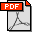H.K. Kim, L.J. Guibas, and S.Y. Shin, Efficient collision detection among moving spheres with unknown trajectories, Algorithmica 43(3), pp. 195-210, 2005. Abstract: Collision detection is critical for applications that demand a great deal of spatial interaction among objects. In such applications the trajectory of an object is often not known in advance either since a user is allowed to move an object at his/her will, or since an object moves under the rules that are hard to describe by exact mathematical formulas. In this paper we present a new algorithm that efficiently detects the collisions among moving spheres with unknown trajectories. We assume that the current position and velocity of every sphere can be probed at any time although its trajectory is unknown. We also assume that the magnitude of the acceleration of each sphere is bounded. Under these assumptions, we represent the bounding volume of the sphere as a moving sphere of variable radius, called a time-varying bound. Whenever the time-varying bounds of two spheres collide with each other, they are checked for actual collision. Exploiting these bounds, the previous event-driven approach for detecting the collisions among multiple moving spheres with ballistic trajectories is generalized for those with unknown trajectories. The proposed algorithm shows an interactive performance for thousands of moving spheres with unknown trajectories without any hardware help. Bibtex: ```@article{kgs-ecdamsut-05, author = "H.K. Kim and L.J. Guibas and S.Y. Shin", title = "Efficient collision detection among moving spheres with unknown trajectories", journal = "Algorithmica", volume =43, numnber =3. pages ="195--210", year =2005 }```